|

# 软装八大风格,总有一款你喜欢!

Top1

崇尚时尚的现代简约风格

现代简约风格，很好地阐述了不拘小节，没有束缚的设计理念。简约明了的线条让空间变的一览无余。也避免了结构复杂和色彩多样带了的审美疲劳。越来越多时尚人士开始推崇这种风格。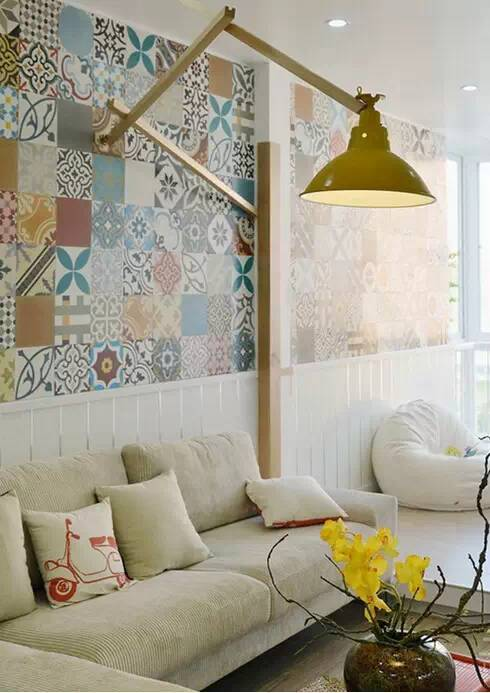Top2

张扬个性的现代前卫

无常规的空间结构，大胆鲜明对比强烈的色彩布置，以及刚柔并济的选材搭配，无不让人在冷峻中寻求到一种超现实的平衡，而这种平衡无疑也是对审美单一、居住理念单一、生活方式单一的最有力的抨击。现代前卫的设计风格在内容和形式上更加出人意料，夺人耳目，常常成为艺术人类的家居设计中的首选。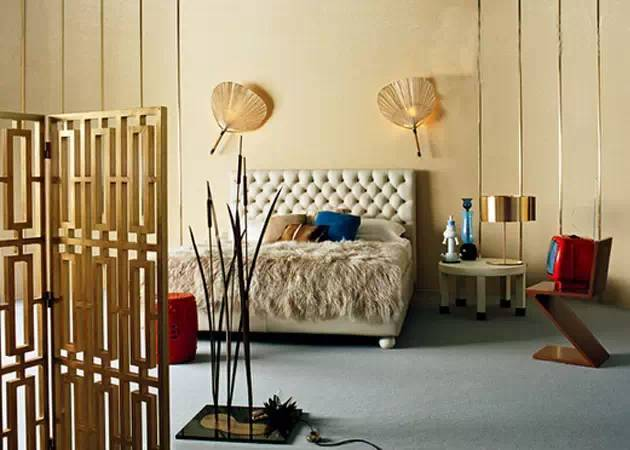Top3

优雅温馨的雅致风格

雅致主义是近几年刚刚兴起又被消费者所迅速接受的一种设计方式，特别是对于文艺界、教育界的朋友来说。如果你喜欢欧式古典的浪漫，却又不想被高贵的繁琐束缚；如果你喜欢简约的干练，但它又不够典雅，缺少温馨，那么不妨尝试雅致主义的设计。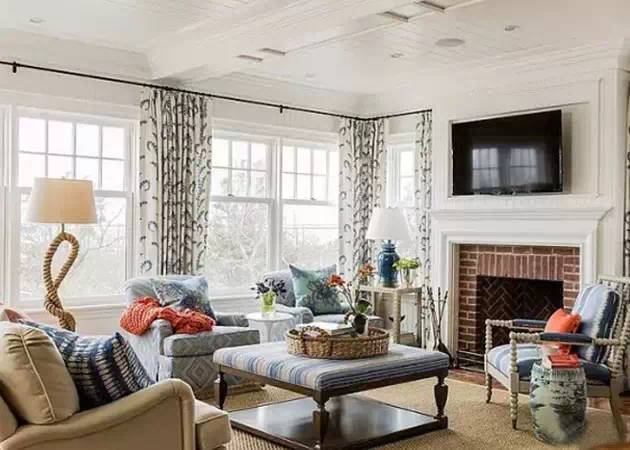Top4

怀旧复古的新中式风格

新中式风格在设计上继承了唐代、明清时期家居理念的精华，将其中的经典元素提炼并加以丰富，同时改变原有布局中等级、尊卑等封建思想，给传统家居文化注入了新的气息。没有刻板却不失庄重，注重品质但免去了不必要的苛刻，这些构成了新中式风格的独特魅力。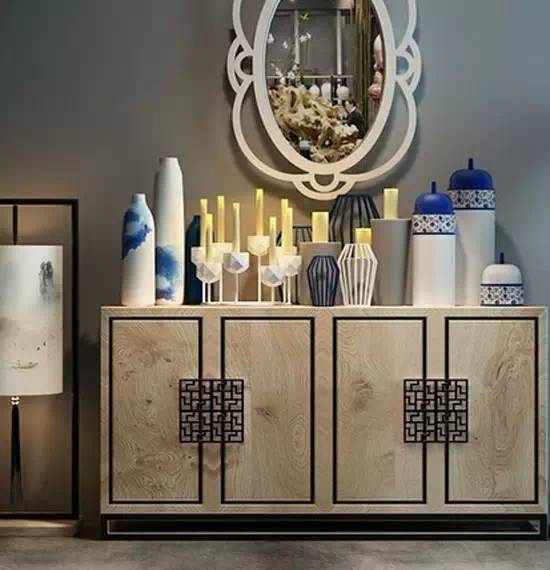Top5

高贵华丽的新古典风格

在注重装饰效果的同时，用现代的手法和材质还原古典气质，新古典风格具备了古典与现代的双重审美效果，完美的结合也让人们在享受物质文明的同时得到了精神上的慰藉。“行散神聚”是新古典风格的主要特点。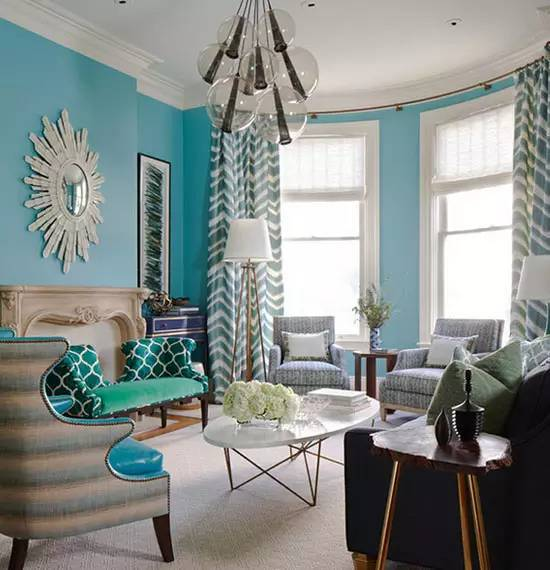Top6

豪华动感的欧式古典风格

作为欧洲文艺复兴时期的产物，古典主义设计风格继承了巴洛克风格中豪华、动感、多变的视觉效果，也吸取了洛可可风格中唯美律动的细节处理元素，受到了社会上层人士的青睐。特别是古典风格中，深沉里显露尊贵、典雅浸透豪华的设计哲学，也成为这些成功人士享受快乐，理念生活的一种写照。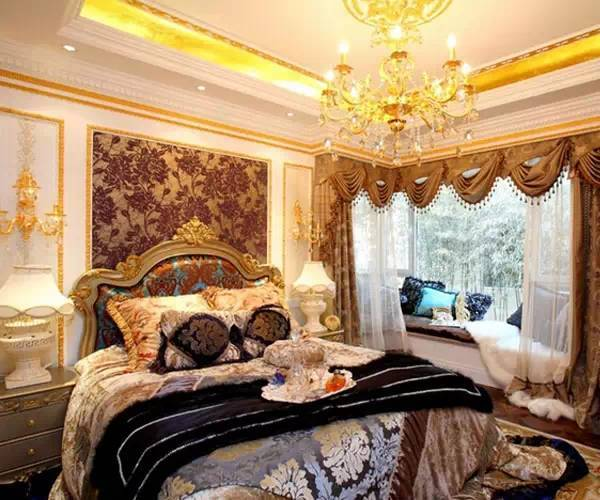Top7

休闲自然的美式乡村风格

美式乡村风格摒弃了繁琐和奢华，并将不同风格中优秀元素汇集融合，以舒适机能为导向，强调“回归自然”，使这种风格变的更加轻松、舒适。美式乡村风格突出了生活的舒适和自由，不论是感觉笨重的家具，还是带有岁月沧桑的配饰，都在告诉人们这一点。特被是在墙面色彩选择上，自然、怀旧、散发着浓郁泥土芬芳的色彩是美式乡村风格的典型特征。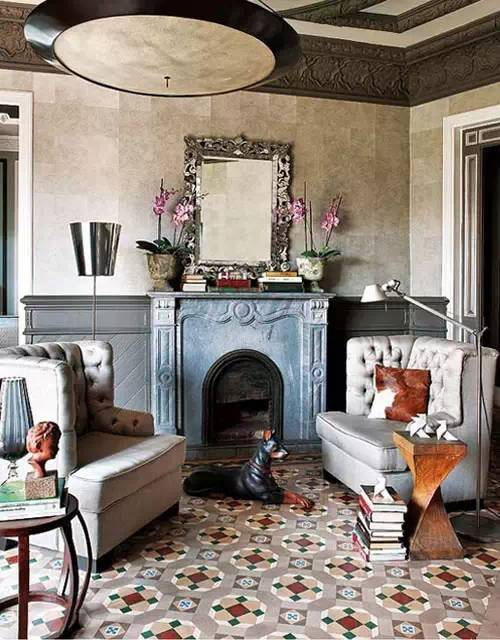Top8

宁静神秘的地中海风格

无处不在的浪漫主义气息和兼容并蓄的文化品位，以其极具亲和力的田园风情，很快被地中海以外的广大区域人群所接受。对于久居都市，习惯了喧嚣的现代都市人而言，地中海风格给人们以返璞归真的感受，同时体现了对于更高生活质量的要求。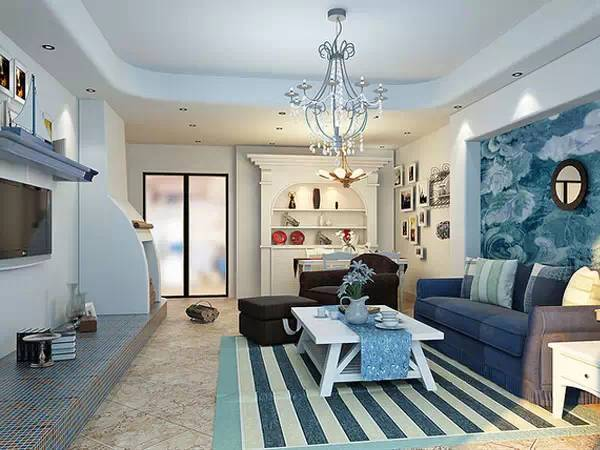什么是软装配饰中的八大元素呢？我们知道整体进行软装配饰设计，可以很好的反应主人家里的生活品质，格调。要进行软装配饰，我们应该从那几个方面着手呢？软装设计八大元素：色彩，家具、布艺、灯具、画品、饰品、花艺、收藏品。做软装配饰从这八大方面考虑就很全面了，也就是我们所说的“八大元素”。

— 八大元素之：色彩—

色彩作为一个奇妙的东西，通过色相、纯度、色调、对比等手段表达人们的情感和联想，从而影响人们的心理和生理反应，甚至影响人们对事物的客观理解和看法，可以说色彩是软装设计的精髓与灵魂。作为软装设计爱好者就要把握好色彩的基础以及其搭配，从而设计出高效的成功作品。

—八大元素之：家具 —

包括支撑类家具、储藏类家具、装饰类家具。如沙发、茶几、床、餐桌、餐椅、书柜、衣柜、电视柜等。

家具作为人们生活、工作必不可少的用具，必须满足人们生活的使用需要，还要满足人们一定的审美要求。在软装设计中，家具的地位至关重要，一个作品的风格基本是由家具主导的，因此，在软装设计中，家具是必备的。当今软装中，家具风格一般分为巴洛克式家具（雄浑壮美）、洛可可式家具（细腻柔美）、美式家具（简明优雅）、现代欧式家具（简洁亲切）、地中海家具（浪漫多姿）、中式家具（高贵且具有收藏价值）、现代家具（浓郁的地域自然色）、现代意大利家具（低调奢华）、船木家具（质朴的艺术）。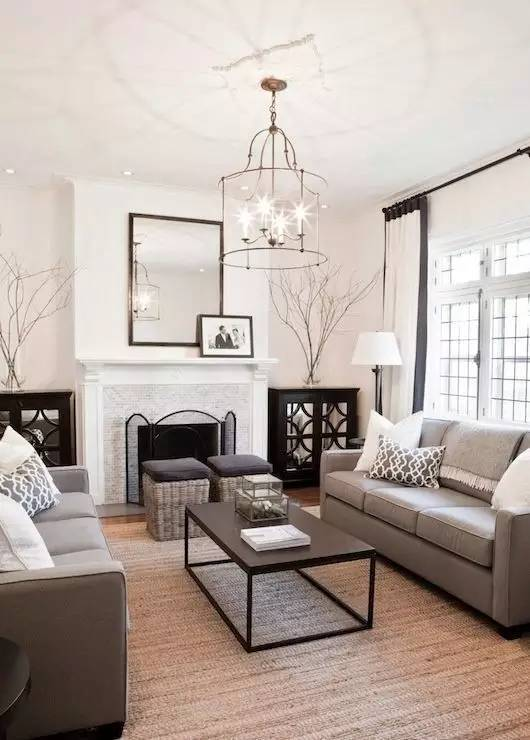—八大元素之：花艺 —

花艺主要是指借助一定的技术与工艺,使花朵的组合变得更加具有美感、形成一种特殊语言、让欣赏者有所感悟的艺术表现形式。现代社会生活节奏不断加快,在这样的背景下,每个人都需要一种温馨舒适的环境来进一步缓解工作带来的疲惫。花艺软装饰这一新兴室内设计元素开始在室内设计中体现出画龙点睛的核心作用。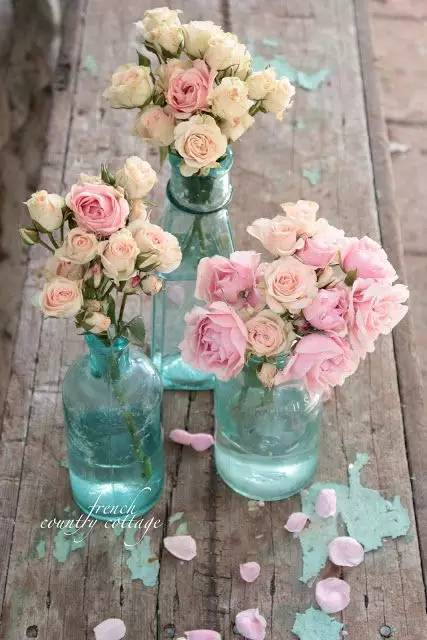—八大元素之：画品 —

装饰品中运用最普遍的要属装饰画。装饰画既要体出功能性的一面——加强房间的功能效果，又要能体现出主人独特的审美观，成为家居人文环境的点睛之笔。—八大元素之：布艺 —

选择布艺不但要考虑与家庭装修风格的搭配,还要符合主人的年纪、性格,年轻的主人不应该选择颜色老成、款式稳重的窗帘布艺,年纪较大的人不宜选用颜色太浅、花纹图案太活泼、款式过于新潮超前的布艺。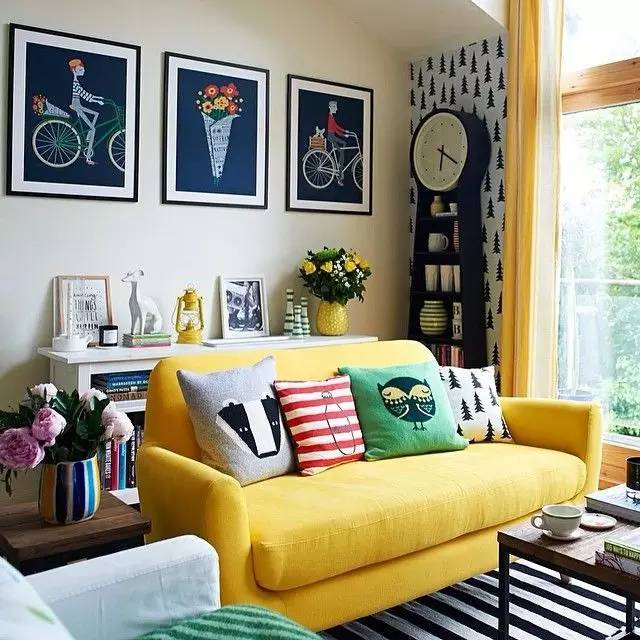—八大元素之：灯具 —

现代软装饰设计中，灯具的作用了除了照明之外，更多的时候起到是装饰作用。虽然灯具变化可谓日新月异，但我们还是从风格上把它分为：中式、欧式、现代、美式以及地中海风格。在室内软装设计中，对于灯具，我们不能随随便便地选择。首先，要具备可观赏性，要求材质优质，造型别致，色彩丰富；其次，要与营造的风格氛围相一致；再者，布光形式要经过精心设计，注重与空间、家具、陈设等配套装饰相协调；最后，还需要突出个性，光源的色彩按用户需要营造出特定的气氛，如热烈、沉稳、安适、宁静、祥和等。面对五彩缤纷、琳琅满目的现代灯具，我们要分清它属于什么类型的软装风格灯具，有什么实用功能和主人的爱好完美结合。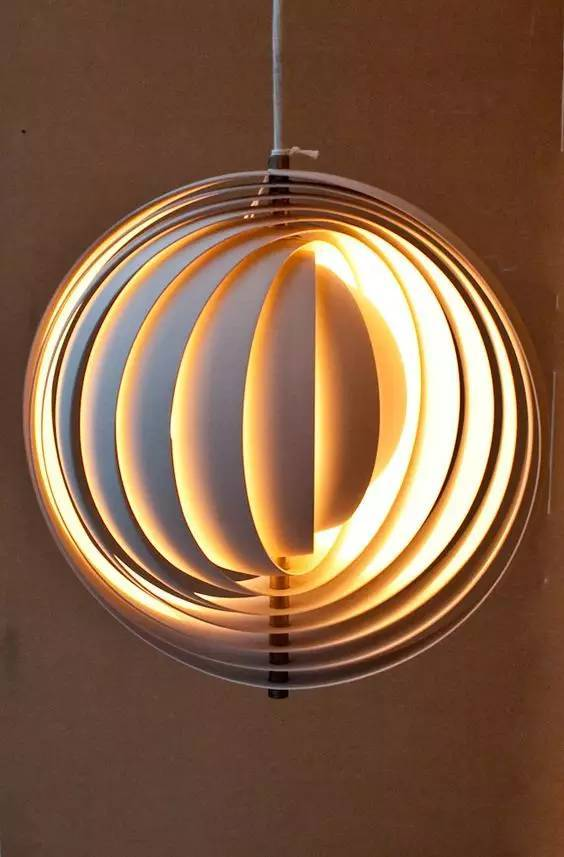—八大元素之：饰品 —

一般为摆件和挂件，包括工艺品摆件、陶瓷摆件、铜制摆件，铁艺摆件，挂画、插画、照片墙、相框、漆画、壁画、装饰画、油画等。

所谓的“画龙点睛”就是说的这个环节，您的家，在彰显品位的同时，是需要很多饰品来映衬的，将细节之美用饰品来体现，这样一个具有完美空间的家就会展现在你的面前！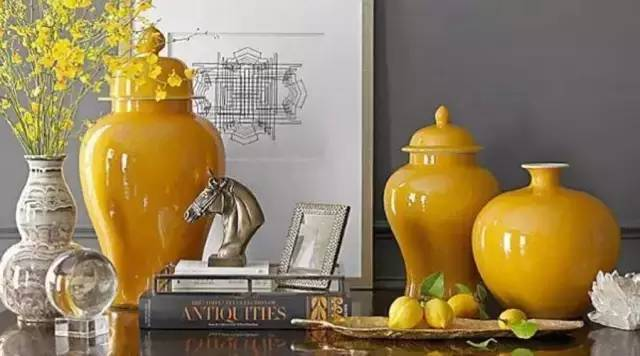—八大元素之：收藏品 —

生活之中，我们肯定会有很多值得我们收藏的点点滴滴。高品质的生活也需要我们来收藏生活之美。`声明：本文由入驻焦点开放平台的作者撰写，除焦点官方账号外，观点仅代表作者本人，不代表焦点立场错误信息举报电话： 400-099-0099，邮箱：jubao@vip.sohu.com，或点此进行意见反馈，或点此进行举报投诉。`A B C D E F G H J K L M N P Q R S T W X Y Z
A - B - C - D - E
• A
• 鞍山
• 安庆
• 安阳
• 安顺
• 安康
• 澳门
• B
• 北京
• 保定
• 包头
• 巴彦淖尔
• 本溪
• 蚌埠
• 亳州
• 滨州
• 北海
• 百色
• 巴中
• 毕节
• 保山
• 宝鸡
• 白银
• 巴州
• C
• 承德
• 沧州
• 长治
• 赤峰
• 朝阳
• 长春
• 常州
• 滁州
• 池州
• 长沙
• 常德
• 郴州
• 潮州
• 崇左
• 重庆
• 成都
• 楚雄
• 昌都
• 慈溪
• 常熟
• D
• 大同
• 大连
• 丹东
• 大庆
• 东营
• 德州
• 东莞
• 德阳
• 达州
• 大理
• 德宏
• 定西
• 儋州
• 东平
• E
• 鄂尔多斯
• 鄂州
• 恩施
F - G - H - I - J
• F
• 抚顺
• 阜新
• 阜阳
• 福州
• 抚州
• 佛山
• 防城港
• G
• 赣州
• 广州
• 桂林
• 贵港
• 广元
• 广安
• 贵阳
• 固原
• H
• 邯郸
• 衡水
• 呼和浩特
• 呼伦贝尔
• 葫芦岛
• 哈尔滨
• 黑河
• 淮安
• 杭州
• 湖州
• 合肥
• 淮南
• 淮北
• 黄山
• 菏泽
• 鹤壁
• 黄石
• 黄冈
• 衡阳
• 怀化
• 惠州
• 河源
• 贺州
• 河池
• 海口
• 红河
• 汉中
• 海东
• 怀来
• I
• J
• 晋中
• 锦州
• 吉林
• 鸡西
• 佳木斯
• 嘉兴
• 金华
• 景德镇
• 九江
• 吉安
• 济南
• 济宁
• 焦作
• 荆门
• 荆州
• 江门
• 揭阳
• 金昌
• 酒泉
• 嘉峪关
K - L - M - N - P
• K
• 开封
• 昆明
• 昆山
• L
• 廊坊
• 临汾
• 辽阳
• 连云港
• 丽水
• 六安
• 龙岩
• 莱芜
• 临沂
• 聊城
• 洛阳
• 漯河
• 娄底
• 柳州
• 来宾
• 泸州
• 乐山
• 六盘水
• 丽江
• 临沧
• 拉萨
• 林芝
• 兰州
• 陇南
• M
• 牡丹江
• 马鞍山
• 茂名
• 梅州
• 绵阳
• 眉山
• N
• 南京
• 南通
• 宁波
• 南平
• 宁德
• 南昌
• 南阳
• 南宁
• 内江
• 南充
• P
• 盘锦
• 莆田
• 平顶山
• 濮阳
• 攀枝花
• 普洱
• 平凉
Q - R - S - T - W
• Q
• 秦皇岛
• 齐齐哈尔
• 衢州
• 泉州
• 青岛
• 清远
• 钦州
• 黔南
• 曲靖
• 庆阳
• R
• 日照
• 日喀则
• S
• 石家庄
• 沈阳
• 双鸭山
• 绥化
• 上海
• 苏州
• 宿迁
• 绍兴
• 宿州
• 三明
• 上饶
• 三门峡
• 商丘
• 十堰
• 随州
• 邵阳
• 韶关
• 深圳
• 汕头
• 汕尾
• 三亚
• 三沙
• 遂宁
• 山南
• 商洛
• 石嘴山
• T
• 天津
• 唐山
• 太原
• 通辽
• 铁岭
• 泰州
• 台州
• 铜陵
• 泰安
• 铜仁
• 铜川
• 天水
• 天门
• W
• 乌海
• 乌兰察布
• 无锡
• 温州
• 芜湖
• 潍坊
• 威海
• 武汉
• 梧州
• 渭南
• 武威
• 吴忠
• 乌鲁木齐
X - Y - Z
• X
• 邢台
• 徐州
• 宣城
• 厦门
• 新乡
• 许昌
• 信阳
• 襄阳
• 孝感
• 咸宁
• 湘潭
• 湘西
• 西双版纳
• 西安
• 咸阳
• 西宁
• 仙桃
• 西昌
• Y
• 运城
• 营口
• 盐城
• 扬州
• 鹰潭
• 宜春
• 烟台
• 宜昌
• 岳阳
• 益阳
• 永州
• 阳江
• 云浮
• 玉林
• 宜宾
• 雅安
• 玉溪
• 延安
• 榆林
• 银川
• Z
• 张家口
• 镇江
• 舟山
• 漳州
• 淄博
• 枣庄
• 郑州
• 周口
• 驻马店
• 株洲
• 张家界
• 珠海
• 湛江
• 肇庆
• 中山
• 自贡
• 资阳
• 遵义
• 昭通
• 张掖
• 中卫

1室1厅1厨1卫1阳台

1
2
3
4
5

0
1
2

1

1

0
1
2
3报名成功，资料已提交审核A B C D E F G H J K L M N P Q R S T W X Y Z
A - B - C - D - E
• A
• 鞍山
• 安庆
• 安阳
• 安顺
• 安康
• 澳门
• B
• 北京
• 保定
• 包头
• 巴彦淖尔
• 本溪
• 蚌埠
• 亳州
• 滨州
• 北海
• 百色
• 巴中
• 毕节
• 保山
• 宝鸡
• 白银
• 巴州
• C
• 承德
• 沧州
• 长治
• 赤峰
• 朝阳
• 长春
• 常州
• 滁州
• 池州
• 长沙
• 常德
• 郴州
• 潮州
• 崇左
• 重庆
• 成都
• 楚雄
• 昌都
• 慈溪
• 常熟
• D
• 大同
• 大连
• 丹东
• 大庆
• 东营
• 德州
• 东莞
• 德阳
• 达州
• 大理
• 德宏
• 定西
• 儋州
• 东平
• E
• 鄂尔多斯
• 鄂州
• 恩施
F - G - H - I - J
• F
• 抚顺
• 阜新
• 阜阳
• 福州
• 抚州
• 佛山
• 防城港
• G
• 赣州
• 广州
• 桂林
• 贵港
• 广元
• 广安
• 贵阳
• 固原
• H
• 邯郸
• 衡水
• 呼和浩特
• 呼伦贝尔
• 葫芦岛
• 哈尔滨
• 黑河
• 淮安
• 杭州
• 湖州
• 合肥
• 淮南
• 淮北
• 黄山
• 菏泽
• 鹤壁
• 黄石
• 黄冈
• 衡阳
• 怀化
• 惠州
• 河源
• 贺州
• 河池
• 海口
• 红河
• 汉中
• 海东
• 怀来
• I
• J
• 晋中
• 锦州
• 吉林
• 鸡西
• 佳木斯
• 嘉兴
• 金华
• 景德镇
• 九江
• 吉安
• 济南
• 济宁
• 焦作
• 荆门
• 荆州
• 江门
• 揭阳
• 金昌
• 酒泉
• 嘉峪关
K - L - M - N - P
• K
• 开封
• 昆明
• 昆山
• L
• 廊坊
• 临汾
• 辽阳
• 连云港
• 丽水
• 六安
• 龙岩
• 莱芜
• 临沂
• 聊城
• 洛阳
• 漯河
• 娄底
• 柳州
• 来宾
• 泸州
• 乐山
• 六盘水
• 丽江
• 临沧
• 拉萨
• 林芝
• 兰州
• 陇南
• M
• 牡丹江
• 马鞍山
• 茂名
• 梅州
• 绵阳
• 眉山
• N
• 南京
• 南通
• 宁波
• 南平
• 宁德
• 南昌
• 南阳
• 南宁
• 内江
• 南充
• P
• 盘锦
• 莆田
• 平顶山
• 濮阳
• 攀枝花
• 普洱
• 平凉
Q - R - S - T - W
• Q
• 秦皇岛
• 齐齐哈尔
• 衢州
• 泉州
• 青岛
• 清远
• 钦州
• 黔南
• 曲靖
• 庆阳
• R
• 日照
• 日喀则
• S
• 石家庄
• 沈阳
• 双鸭山
• 绥化
• 上海
• 苏州
• 宿迁
• 绍兴
• 宿州
• 三明
• 上饶
• 三门峡
• 商丘
• 十堰
• 随州
• 邵阳
• 韶关
• 深圳
• 汕头
• 汕尾
• 三亚
• 三沙
• 遂宁
• 山南
• 商洛
• 石嘴山
• T
• 天津
• 唐山
• 太原
• 通辽
• 铁岭
• 泰州
• 台州
• 铜陵
• 泰安
• 铜仁
• 铜川
• 天水
• 天门
• W
• 乌海
• 乌兰察布
• 无锡
• 温州
• 芜湖
• 潍坊
• 威海
• 武汉
• 梧州
• 渭南
• 武威
• 吴忠
• 乌鲁木齐
X - Y - Z
• X
• 邢台
• 徐州
• 宣城
• 厦门
• 新乡
• 许昌
• 信阳
• 襄阳
• 孝感
• 咸宁
• 湘潭
• 湘西
• 西双版纳
• 西安
• 咸阳
• 西宁
• 仙桃
• 西昌
• Y
• 运城
• 营口
• 盐城
• 扬州
• 鹰潭
• 宜春
• 烟台
• 宜昌
• 岳阳
• 益阳
• 永州
• 阳江
• 云浮
• 玉林
• 宜宾
• 雅安
• 玉溪
• 延安
• 榆林
• 银川
• Z
• 张家口
• 镇江
• 舟山
• 漳州
• 淄博
• 枣庄
• 郑州
• 周口
• 驻马店
• 株洲
• 张家界
• 珠海
• 湛江
• 肇庆
• 中山
• 自贡
• 资阳
• 遵义
• 昭通
• 张掖
• 中卫• 手机• 分享
• 设计
免费设计
• 计算器
装修计算器
• 入驻
合作入驻
• 联系
联系我们
• 置顶
返回顶部